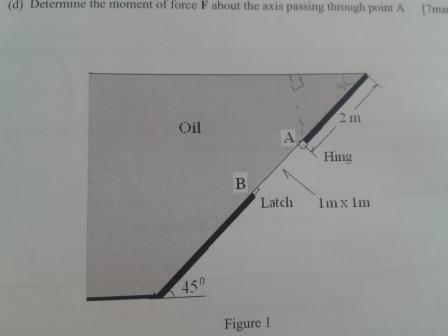# Fluid mechanics civil engineering

Thread moved from the technical forums, so no HH Template is shown.As shown in the attached image, a tank has an inclined wall at an angle of 450 to the horizontal. On this wall, there is a 1m square door that is hinged at A and has a simple latch at B. The distance between A and the free surface of sea water measured along the inclined wall is 2 m. (Specific gravity of sea water sg = 1.1). Calculate the force F on the door due to the oil
MY WORKING OUT could anyone pls help check if i am right :) P=F/A SO F=PA than F=densitygravitysin(angle)Areaheight now in order to find the height cause it's inclined i did sin 90/2=sin45/x cross multiply to get x-1.414 back to the formula 1100*9.81*sin45*1m^2*1.414 and i got 10.789kN as my force

BvU
Hello Mikee,